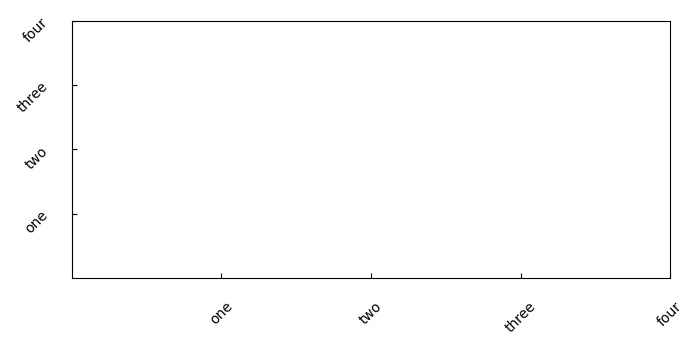# How to display all label values in Matplotlib?

To display all label values, we can use set_xticklabels() and set_yticklabels() methods.

## Steps

• Create a list of numbers (x) that can be used to tick the axes.

• Get the axis using subplot() that helps to add a subplot to the current figure.

• Set the ticks on X and Y axes using set_xticks and set_yticks methods respectively and list x (from step 1).

• Set tick labels with label lists (["one", "two", "three", "four"]) and rotation of 45 using set_xticklabels() and set_yticklabels().

• To add space between axes and tick labels, we can use tick_params() method with pad argument that helps to add space. Argument direction (in) helps to put ticks inside the axes, and axis (both), parameters to be applied on both the axes.

• To show the figure, use plt.show() method.

## Example

import matplotlib.pyplot as plt
plt.rcParams["figure.figsize"] = [7.00, 3.50]
plt.rcParams["figure.autolayout"] = True
x = [1, 2, 3, 4]
ax1 = plt.subplot()
ax1.set_xticks(x)
ax1.set_yticks(x)
ax1.set_xticklabels(["one", "two", "three", "four"], rotation=45)
ax1.set_yticklabels(["one", "two", "three", "four"], rotation=45)
plt.show()